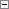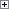Indicates whether a C/AL variant contains a Time variable.

Ok := Variant.ISTIME

Variant

Type: VariantProperty Value/Return Value

Type: Boolean

true if the C/AL variant contains a Time variable; otherwise, false.Example

The following example determines whether a C/AL variant contains a time variable. The code initializes the MyTime variable with a Time value. The MyTime variable is assigned to the variant variable that is named MyVariant. The ISTIME function determines whether the variant contains a Time variable and stores the return value in the varResult variable. In this case, the variant contains a Time variable so Yes is returned and displayed in a message box. The ISCODE Function (Variant) determines whether the variant contains a Code variable. The return value is No because the variant does not contain a code. This example requires that you create the following variables and text constants in the C/AL Globals window.

Variable name DataType

MyTime

Time

MyVariant

Variant

varResult

Boolean

Text constant name Enu value

Text000

Does the variant >%1< contain a time variable? %2.

Text001

Does the variant >%1< contain a code variable? %2.Copy Code
MyTime := TIME;
MyVariant := MyTime;
varResult := MyVariant.ISTIME;
MESSAGE(Text000,MyVariant,varResult);
varResult := MyVariant.ISCODE;
MESSAGE(Text001,MyVariant,varResult);See Also

Reference

Variant Data Type